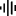# Signal Processing (AI.Associates)

DSP

This category contains 7 nodes.

##### Fast Fourier Transform (FFT)

This node performs a forward Fast Fourier Transformation(FFT) on each row of the input table. Generally speaking, it extracts the frequencies of an input […]

##### Frequency Domain Features (FDF)

This node computes statistics in the frequency domain. The chosen statistics will be computed for each row.

##### Time Domain Features (TDF)

This node computes statistics in the time domain. The chosen statistics will be computed for each row.

This node reads in .wav files.

##### Welch Averaging

This node bundles together a number of rows and takes an average in every column for this bundle.

##### Window Function

This node multiplies the input rows with a window function to prevent spectral leakage in subsequent FFT.

##### Window Slider

This node creates sliding windows from an input signal which is stored in a table with one column.